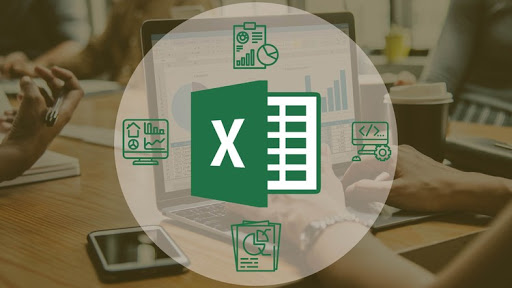A complete Excel course, so that you excel in all important topics with Excel 2016, Excel 2013, Excel 2010 & Excel 2007
Instructed by: Start-Tech Academy | Subject: Office Productivity , MicrosoftMicrosoft Excel is easy but no one can determine the learning time it takes. It totally depends on you. The method we adopted to help you learn Microsoft Excel quickly starts from the basics and takes you to advanced level within hours. You can follow the same, but remember you can learn nothing without practicing it. Practice is the only way to learn Microsoft Excel quickly.

What are the steps I should follow to learn Microsoft Excel? 1. Start learning from the basics of Microsoft Excel. The first 3 sections of the course cover the basics. 2. Once done with the basic try your hands on advanced MS Excel. Next 7 sections cover Advanced Excel topics 3. Next section will help you some cool new tricks of Microsoft Excel. 4. Practice your learning on the exercise provided with every lecture. What are the topics covered in this course? Below are the Excel course contents of this complete and concise course on Microsoft Excel: Introduction - In this video, the structure and contents of the course are discussed.

Mathematical Functions - This Excel lecture covers Mathematical formulas such as SUM, AVERAGE,RAND, MIN & MAX, SUMPRODUCT. Textual Formulas - This Excel lecture covers Textual formulas such as TRIM, CONCATENATE, SUBSTITUTE, UPPER & LOWER, LENGTH, LEFT, RIGHT & MID Logical Formulas - This Excel lecture covers Logical formulas such as AND & OR, IF, COUNTIF, SUMIF Date-time (Temporal) Formulas - This Excel lecture covers Date-time related functions such as TODAY & NOW, DAY, MONTH & YEAR, DATEDIF & DAYS Lookup Formulas - This Excel lecture covers Lookup formulas such as VLOOKUP, HLOOKUP, INDEX, MATCH Data Tools - This Excel lecture covers Data operating tools such as Data Sorting and Filtering, Data validation, Removing duplicates, Importing Data (Text-to-columns) Formatting data and tables - This Excel lecture covers data formatting options such as coloring, changing font, alignments and table formatting options such as adding borders, having highlighted table headers, banded rows etc..

Pivot Tables - This Excel lecture covers Pivot tables end-to-end. Charts - This Excel lecture covers charts such as, Bar/ Column chart, Line Chart, Scatter Plot, Pie & Doughnut charts, Statistical Chart - Histogram, Waterfall, Spartlines Excel Shortcuts - This Excel lecture will introduce you to some important shortcuts and teach you how to find out the shortcut for any particular excel operation. Analytics in Excel - This Excel lecture covers the data analytics options available in Excel such as Regression, Solving linear programming problem (Minimization or Maximization problems), What-if (Goal Seek and Scenario Manager) Macros - This Excel lecture covers the process of recording a Macro, running a Macro and creating a button to run a Macro. Bonus Lectures - Waterfall chart in Excel 2016 and previous versions of Excel, Infographics 1: Cool charts, Infographics 2: Cool charts

What is the difference between basic and advanced level of Excel?

At Basic level of MS Excel a person Can build excel formulas using: SUM, IF, AVERAGE, COUNT, ROUND Is comfortable building excel formulas to manipulate text and dates Understands and can use the Filter and Sort feature of Microsoft Excel. Can create basic charts like Line chart, bar chart and pie chart At Advanced level of MS Excel a person Can implement Excel LOOKUP Formulas like VLOOKUP, HLOOKUP, Index and Match Can use conditional and logical formulas like IF, SUMIF, COUNTIF, OR, AND etc. Knows what a Pivot Table is and how to build one. Knows what an add-in is and how to install one. Can record a macro and use it later. Can successfully edit/modify simple recorded macros. Can create advanced charts like Waterfall chart and overlay chart in Microsoft Excel Can create solve analytics problem using excel solver.

We have created a set of concise and comprehensive videos to teach you all the Excel related skills you will need in your professional career. Do With each lecture, we have provide a practice sheet to complement the learning in the lecture video. These sheets are carefully designed to further clarify the concepts and help you with implementing the concepts on practical problems faced on-the-job. Review Check if you have learnt the concepts by comparing your solutions provided by us. Ask questions in the discussion board if you face any difficulty. Apart from this, explore fun and exciting use cases of Excel in our bonus videos. The Authors of this course have several years of corporate experience and hence have curated the course material keeping in mind the requirement of Excel in today's corporate world.

### Course Info

Excel Masterclass: Complete and Concise Microsoft ExcelReviewed by Acamig Courses on April 11, 2019 Rating: 5